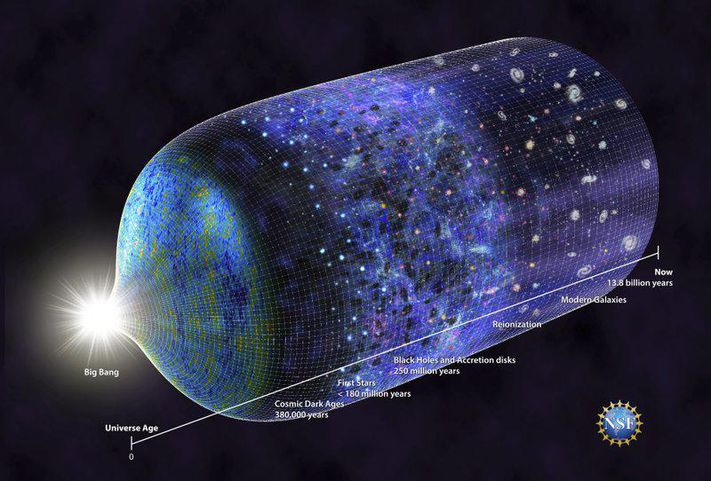## Multiply Connected UniversesIs the cube a hexahedron? From a topological point of view, the shape of space depends on its overall surface.

Thus, the ad-hoc projection of a cube on a plane actually draws a hexagon, because the cube is a multiply connected space whose faces are identified two-by-two according to certain geometric transformations.

In this context, we can ask the question: might the universe be a multiply connected space? If so, we should observe phantom images of celestial bodies in different directions (these are not gravitational illusions).

We would not be able to distinguish them from the real objects because their brilliance, shape and stage of development could be different depending on the resulting “projection”.

The universe could therefore have a very stretched spatial dimension. But this would be an illusion caused by the multiplied reflections of a multiply connected world reduced to a few million light years.

To date, within the local supercluster there are no phantom images of neighboring galaxies, but we are unable to state that in spatio-temporal infinitude the universe is not multiply connected.

Since this concept poses problems for mathematicians as well as physicists, they try to eliminate the concept of infinity from their theories.